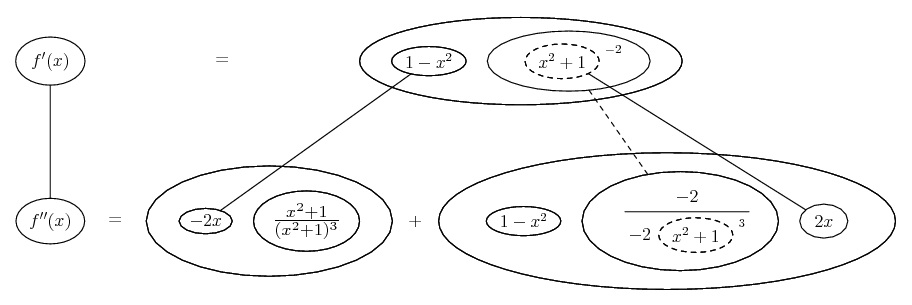# Thread: Second derivative help

1. ## Second derivative help

I have this practice problem and we were told to find the derivative of

$\displaystyle f(x)=\frac{x}{x^2+1}$

which is $\displaystyle f\prime(x)=\frac{1-x^2}{(x^2+1)^2}$

We didn't have to find the second derivative but our text gave us the answer to it.

$\displaystyle f\prime\prime(x)=\frac{(x^2+1)^2(-2x)-(1-x^2)(2)(1+x^2)(2x)}{(x^2+1)^4}=\frac{(2x)(x^2-3)}{(x^2+1)^3}$

So my question is what cancels out in the numerator to arrive at the answer. It's something so elementary but I can't seem to get my answer to match the final answer.

2. $\displaystyle f^{\prime\prime}(x)=\frac{(x^2+1)^2(-2x)-(1-x^2)(2)(1+x^2)(2x)}{(x^2+1)^4}=$

$\displaystyle (x^2+1)\frac{-2x(x^2+1)-4x(1-x^2)}{(x^2+1)^4} ={-2x(x^2+1 +2(1-x^2)) \over (x^2+1)^3} =$

$\displaystyle {-2x(-x^2 + 3) \over (x^2+1)^3} = \frac{(2x)(x^2-3)}{(x^2+1)^3}$

3.Originally Posted by ascendancy523I have this practice problem and we were told to find the derivative of

$\displaystyle f(x)=\frac{x}{x^2+1}$

which is $\displaystyle f\prime(x)=\frac{1-x^2}{(x^2+1)^2}$

We didn't have to find the second derivative but our text gave us the answer to it.

$\displaystyle f\prime\prime(x)=\frac{(x^2+1)^2(-2x)-(1-x^2)(2)(1+x^2)(2x)}{(x^2+1)^4}$

So my question is what cancels out in the numerator to arrive at the answer. It's something so elementary but I can't seem to get my answer to match the final answer.

$\displaystyle \frac{(x^2+1)^2(-2x)-(1-x^2)(2)(1+x^2)(2x)}{(x^2+1)^4}=\frac{(2x)(x^2-3)}{(x^2+1)^3}$

note the common factors in the numerator's two terms ...

$\displaystyle \frac{(x^2+1)(-2x)\left[(x^2+1) + 2(1-x^2)\right]}{(x^2+1)^4}$

$\displaystyle \frac{(-2x)\left[(x^2+1) + 2(1-x^2)\right]}{(x^2+1)^3}$

$\displaystyle \frac{(-2x)\left(3-x^2\right)}{(x^2+1)^3}$

$\displaystyle \frac{(2x)\left(x^2-3\right)}{(x^2+1)^3}$

4. However... quicker by balloon!

At any rate, you don't need the quotient rule, or raising of the bottom power to 4.... where... is the chain rule, wrapped in...... the product rule. Straight continuous lines differentiate downwards (integrate up) with respect to x, and the straight dashed line similarly but with respect to the dashed balloon expression (which is the inner function of the composite and hence subject to the chain rule).

__________________________________________
Don't integrate - balloontegrate!

Balloon Calculus: Standard Integrals, Derivatives and Methods

Balloon Calculus Drawing with LaTeX and Asymptote!

#### Search Tags

derivative